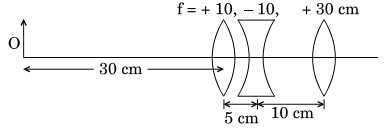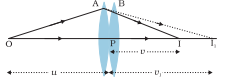# (a) Using the ray diagram for a system of two lenses of focal lengths and  in contact with each other, show that the two lens system can be regarded as equivalent to a single lens of focal length f, where . Also write the relation for the equivalent power of the lens combination.(b) Determine the position of the image formed by the lens combination given in the figure.(a)   Let us consider the two thin lenses A and B forming the image  and  respectively, from the object (O) For I the distance is u  and for  the distance isFor lens 'A', the lens formula is ;

_______(1)

Where v is the image distance

u is the object distance

For lens 'B' , the lens formula is :

________(2)

On adding both equations, we get

then,

Therefore, the equivalent power form here would be

(b)  The image formed by the lense of

This image formed by the lens act as an object from the concave lens.

Therefore,

here,

therefore, virtual image forms at the right of the concave lens at  and act as an object for convex lens

### Preparation Products

##### Knockout NEET Sept 2020

An exhaustive E-learning program for the complete preparation of NEET..

₹ 15999/- ₹ 6999/-
##### Rank Booster NEET 2020

This course will help student to be better prepared and study in the right direction for NEET..

₹ 9999/- ₹ 4999/-
##### Knockout JEE Main Sept 2020

An exhaustive E-learning program for the complete preparation of JEE Main..

₹ 12999/- ₹ 6999/-
##### Test Series NEET Sept 2020

Take chapter-wise, subject-wise and Complete syllabus mock tests and get in depth analysis of your test..

₹ 4999/- ₹ 2999/-